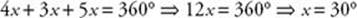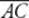﻿ ﻿Exercises - Circles - PLANE GEOMETRY - SAT SUBJECT TEST MATH LEVEL 1

## PLANE GEOMETRY## CHAPTER 11Circles### Exercises1. In the figure above, if O is the center of the circle, what is the value of w?

(A) 21

(B) 38

(C) 42

(D) 69

(E) 1112. In the figure above, if O is the center of the circle, what is m∠C?

(A) 40°

(B) 50°

(C) 80°

(D) 100°

(E) It cannot be determined from the given information.

Questions 3 and 4 refer to the following figure:3. What is the length of arc?

(A) 6

(B) 12

(C) 6π

(D) 12π

(E) 18π

4. What is the area of the shaded sector?

(A) 12

(B) 18

(C) 12π

(D) 18π

(E) 30π

5. What is the area of a circle whose circumference is?

(A)(B)(C)(D) π

(E) 4π

6. What is the circumference of a circle whose area is 100π?

(A) 10

(B) 20

(C) 10π

(D) 20π

(E) 25πNote: Figure not drawn to scale.

7. In the figure above, the ratio of the measure of arcto the measure of arcto the measure of arcis 4 to 3 to 5. What is mA?

(A) 30°

(B) 45°

(C) 60°

(D) 75°

(E) 90°

8. A square of area 2 is inscribed in a circle. What is the area of the circle?

(A)(B)(C) π

(D) π(E) 2π

9. What is the area of a circle that is inscribed in a square of area 2?

(A)(B)(C) π

(D) π(E) 2π

10. If A is the area and C is the circumference of a circle, which of the following is an expression for C in terms of A ?

(A)(B)(C)(D)(E)11. In the figure above, triangle ABC is inscribed in a semicircle. What is the area of the shaded region?

(A) 15.4

(B) 48.6

(C) 71.4

(D) 93.7

(E) 109.7Note: Figure not drawn to scale.

12. In the figure above,is tangent to circle O at point P. If OS = 20 and PS = 12, what is QS?

(A) 4

(B) 8

(C) 9

(D) 10

(E) 11Note: Figure not drawn to scale

13. In the figure above,andare tangents to circle O and the measure of arcis 250°. What is mPAB?

(A) 35°

(B) 55°

(C) 70°

(D) 90°

(E) 110°

14. The circumference of a circle is xπ units, and the area of the circle is yπ square units. If y = 2x, what is the radius of the circle?

(A) 1

(B) 2

(C) 4

(D) 6

(E) 815. The figure above shows a regular hexagon whose sides are 10 inscribed in a circle. What is the area of the shaded region, rounded to the nearest whole number?

(A) 24

(B) 34

(C) 54

(D) 64

(E) 164

 1. (A) 6. (D) 11. (A) 2. (B) 7. (B) 12. (A) 3. (C) 8. (C) 13. (B) 4. (E) 9. (B) 14. (C) 5. (A) 10. (E) 15. (C)

Solutions

Each of the problems in this set of exercises is typical of a question you could see on a Math 1 test. When you take the model tests in this book and, in particular, when you take the actual Math 1 test, if you get stuck on questions such as these, you do not have to leave them out—you can almost always answer them by using one or more of the strategies discussed in the “Tactics” chapter. The solutions given here do not depend on those strategies; they are the correct mathematical ones.

See Important Tactics for an explanation of the symbol ⇒, which is used in several answer explanations.

1. (A) m∠M = m∠N + 138 = 180 ⇒ m∠M = m∠N = 42. Sinceand m∠M = m∠N = w are radii, OM = ON, ΔOMN is isosceles, and m∠M = m∠N = w. Therefore, 2w = 42w = 21.

2. (B) SinceAOB is a central angle, m= 100°. SinceACB is an inscribed angle, m.3. (C) The length of arcisof the circumference, which is 2π(10) = 20π. So the length of arc.

4. (E) The area of the shaded sector isof the area of the circle, which is π(10)2 = 100π. So the area of the shaded sector is.

5. (A)6. (D)7. (B) The sum of the measures of the three arcs is 360°. So:So the measure of arcis 330° = 90°, and m.

8. (C) Draw a picture. Since the area of square ABCD is 2, AD =. So diagonal BD == 2.is also a diameter of the circle. So the diameter is 2 and the radius is 1. Therefore, the area is π(1)2 = π.9. (B) Draw a picture. Since the area of square ABCD is 2, AD =.Then diameter EF =radiusthe area of circle O is.

10. (E)11. (A) Since an angle inscribed in a semicircle is a right angle, ΔABC is a right triangle. By the Pythagorean theorem:Sinceis a diameter, the radius isand the area of the semicircle is. The area of right triangle ABC is.

So the area of the shaded region is.

12. (A) Since, ΔOPS is a right triangle. By the Pythagorean theorem:
OP2 + PS 2 = OS2OP2 + 144 = 400 and so OP2 = 256OP = 16Since all radii are equal, OQ = 16QS = 20 – 16 = 4.13. (B) The measure of the smaller arc from A to B is 360° – 250° = 110°. So m. Since the two tangents are congruent, ΔPAB is isosceles and the measure of each base angle is.

14. (C) Since C = xπ, we have xπ = 2πrx = 2r. Since A = yπ, we have yπ = πr2y = r2. Finally, since it is given that y = 2x,

y = 2xr 2 = 2(2r) = 4rr = 4.

15. (C) Three diameters divide the hexagon into 6 equilateral triangles.By using the formula for the area of an equilateral triangle, we find that the area of the hexagon is. Since the radius of the circle is 10, its area is π(102) = 100π. So the area of the shaded region is.

﻿